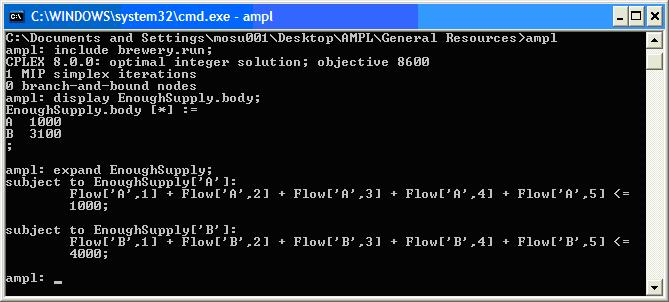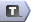# Constraints in AMPL

## Description

Mathematical programmes use constraints to describe the relationships that define a feasible set of decision variables.

## Defining a Constraint

Constraints are defined using the `subject to` keywords, a label and possibly and indexing set, the `:` operator, two expressions and a relational operator:

```subject to <conname> [{conset}] :
<lhs_expression> <relational operator> <rhs_expression>;
```

### Examples

```subject to LimitedResin:
sum {s in SURFBOARDS} Resin[s] * Production[s] <= TotalResin;

# Must conserve flow in the network (steel cannot disappear!)
subject to ConserveFlow {n in NODES}:
sum {m in NODES: (m, n) in ARCS} Shipment[m, n] + Supply[n] =
sum {p in NODES: (n, p) in ARCS} Shipment[n, p] + Demand[n] + Storage[n];
```

## Bounded Constraints

AMPL also allows bounded constraints to be defined. These constraints consist of an expression and a lower and upper bound. The lower and upper bounds may also be expressions, but may only involve parameters. (Note Regular constraints may have variables in either the left- or right-hand-side expressions.)
```subject to MeetRequirement {r in REQUIREMENTS}:
Min[r] <= sum {i in INGREDIENTS} Contributes[i, r] * Amount[i] <= Max[r];
```

## Examining Constraints

We have already seen how to observe the value of variables using display <varname>;. For constraints, we can look at the value of the expression involving the variables (known as the body) by using

```display <conname>.body;
```

For bound constraints this represents the expression in the middle, for other constraints this represents the value of the constraint expression with all variables shifted to the left-hand side. You can see the constraints in modified form by using

```expand <conname>;
```## Changing Constraints

Once constraints have been added to a model you cannot remove them. However, you can drop a given constraint from the model. To drop a constraint, use the `drop` keyword and the constraint name.

```drop <conname>;
```
If you decide to reinstate the constraint, you can restore it. To restore a constraint, use the `restore` keyword and the constraint name.
```restore <conname>;
```

-- MichaelOSullivan - 02 Mar 2008

Topic revision: r1 - 2008-03-02 - MichaelOSullivanCopyright © 2008-2023 by the contributing authors. All material on this collaboration platform is the property of the contributing authors.
Ideas, requests, problems regarding TWiki? Send feedback